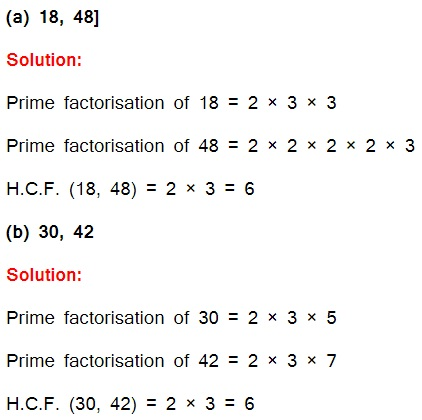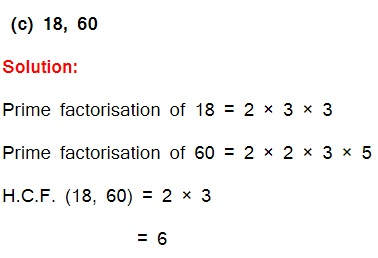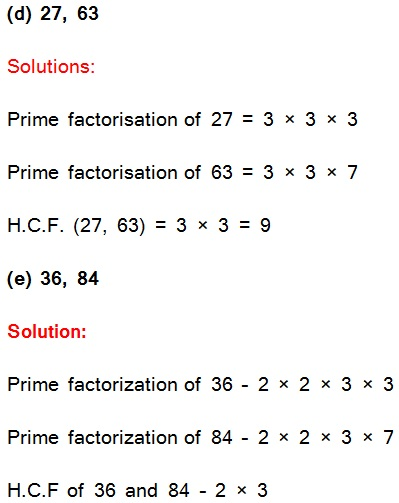Study Materials

# NCERT Solutions for Class 6th Mathematics

Page 6 of 7

## Chapter 3. Playing with Numbers

### Exercise 3.6

Exercise -3.6

Q1. Find the H.C.F. of the following numbers:(f) 34, 102

Solutions:

Prime factorization of 34

= 2 × 17

Prime factorization of 102

= 2 × 3 × 17

HCF of 34 and 102 is 2 × 17

= 34

(g) 70, 105, 175

Solution:

Prime factorization of 70

= 2 × 5 × 7

Prime factorization of 105

= 3 × 5 × 7

Prime factorization of 175

= 5 × 5 × 7

H.C.F. (70, 105, 175)

= 5 × 7

= 35

(h) 91, 112, 49

Solution:

Prime factorization of 91

= 7 × 13

Prime factorization of 112

= 2 × 2 × 2 × 2 × 7

Prime factorization of 49

= 7 × 7

H.C.F. (91, 112, 49) = 7

(i) 18, 54, 81

Solution:

Prime factorization of 18

= 2 × 3 × 3

Prime factorization of 54

= 2 × 3 × 3 × 3

Prime factorization of 81

= 3 × 3 × 3 × 3

H.C.F. = 3 × 3 = 9

(j) 12, 45, 75

Solution:

Prime factorization of 12

= 2 × 2 × 3

Prime factorization of 45

= 3 × 3 × 5

Prime factorization of 75

= 3 x 5 x 5

H.C.F. = 1 × 3

= 3

Q2. What is the H.C.F. of two consecutive:

(a) numbers?

(b) even numbers?

(c) odd numbers?

Solution:

(a) H.C.F. of two consecutive numbers be 1.

(b) H.C.F. of two consecutive even numbers be 2.

(c) H.C.F. of two consecutive odd numbers be 1.

Q3. H.C.F. of co-prime numbers 4 and 15 was found as follows by factorization:

4 = 2 × 2 and

15 = 3 × 5 since there is no common prime factor, so H.C.F. of 4 and 15 is 0. Is the answer correct? If not, what is the correct H.C.F.?

Solution: No. The correct H.C.F. is 1.

Page 6 of 7

Chapter Contents: